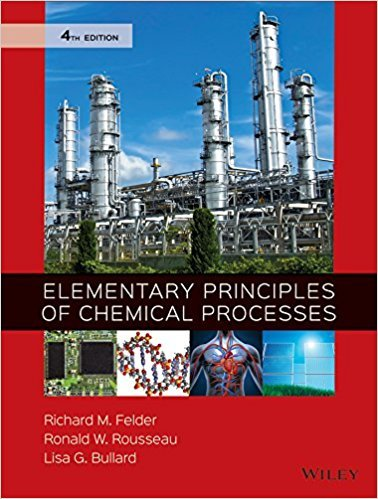×
×

# Solutions for Chapter A.1: THE METHOD OF LEAST SQUARES## Full solutions for Elementary Principles of Chemical Processes | 4th Edition

ISBN: 9780470616291Solutions for Chapter A.1: THE METHOD OF LEAST SQUARES

Solutions for Chapter A.1
4 5 0 396 Reviews
16
0
##### ISBN: 9780470616291

This expansive textbook survival guide covers the following chapters and their solutions. Since 4 problems in chapter A.1: THE METHOD OF LEAST SQUARES have been answered, more than 41743 students have viewed full step-by-step solutions from this chapter. This textbook survival guide was created for the textbook: Elementary Principles of Chemical Processes, edition: 4. Elementary Principles of Chemical Processes was written by and is associated to the ISBN: 9780470616291. Chapter A.1: THE METHOD OF LEAST SQUARES includes 4 full step-by-step solutions.

Key Chemistry Terms and definitions covered in this textbook
• alpha (a) rays.

Helium ions with a positive charge of 12. (2.2)

• atom.

The basic unit of an element that can enter into chemical combination. (2.2)

• cation

A positively charged ion. (Section 2.7)

• chirality center

A tetrahedral carbon atom bearing four different groups.

• Chlorofl uorocarbons (CFCs, Freons)

Compounds with one or two carbons, chlorine, and fl uorine, formerly used as refrigerants

• diamagnetic anisotropy

An effect that causes different regions of space to be characterized by different magnetic field strengths.

• divalent

An element that forms two bonds, such as oxygen.

• doping

Incorporation of a hetero atom into a solid to change its electrical properties. For example, incorporation of P into Si. (Section 12.7)

• electromotive force (emf)

A measure of the driving force, or electrical pressure, for the completion of an electrochemical reaction. Electromotive force is measured in volts: 1 V = 1 J>C. Also called the cell potential. (Section 20.4)

• electron-domain geometry

The three- dimensional arrangement of the electron domains around an atom according to the VSEPR model. (Section 9.2)

• empirical formula

A chemical formula that shows the kinds of atoms and their relative numbers in a substance in the smallest possible whole-number ratios. (Section 2.6)

• Epoxide

A cyclic ether in which oxygen is one atom of a three-membered ring

A substance with one or more unpaired electrons. (Section 21.9)

• osmotic pressure

The pressure that must be applied to a solution to stop osmosis from pure solvent into the solution. (Section 13.5)

• periplanar

A conformation in which a hydrogen atom and a leaving group are approximately coplanar.

• Primary structure of nucleic acids

The sequence of bases along the pentose-phosphodiester backbone of a DNA or RNA molecule read from the 5’ end to the 3’ end

A chemical entity with an unpaired electron.

• spin-spin splitting

A phenomenon observed most commonly for nonequivalent protons connected to adjacent carbon atoms, in which the multiplicity of each signal is affected by the other.

• unsymmetrical ether

An ether(R!O!R) where the two R groups are notidentical.

×